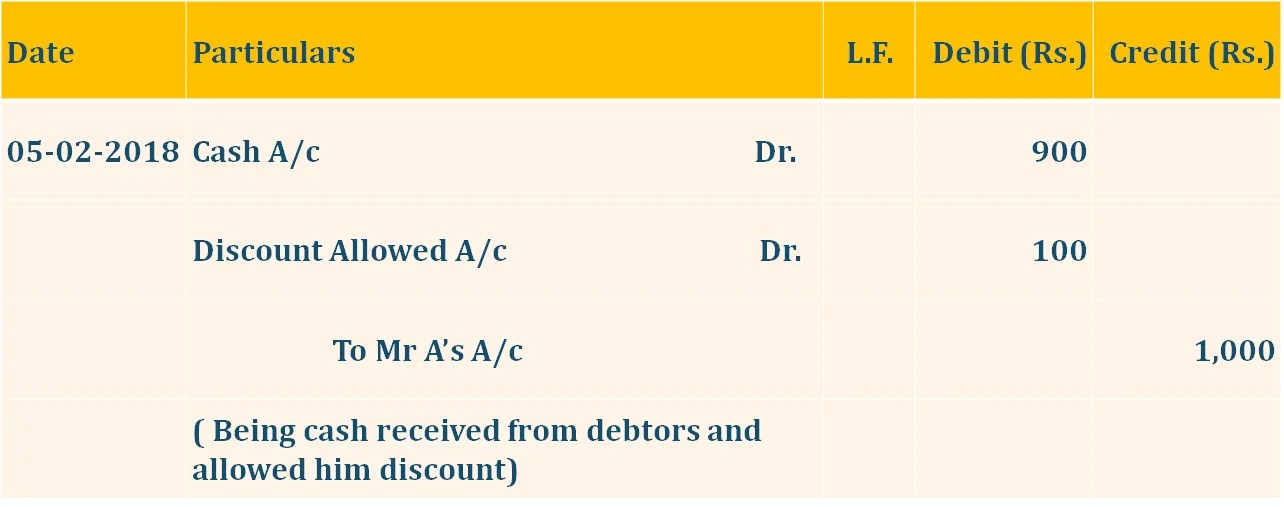# Journal Entry for Discount Allowed | Examples | JournalBefore passing Journal Entry for Discount Allowed, we have to know about the meaning of discount and its type.

### Meaning:

The discount Allowed means the reduction in the selling price of the product.So, it is the loss of the seller of the goods according to Nominal rule he will debit the discount A/c.

For Example, the Sale price of the product is Rs 1,500/- but Retailer offer to the customer only for Rs. 1,350/- after deducting 10% discount. here the amount of discount is Rs 150/-.

Click on the topic name to Jump directly to Topic you want to Read:

## Now, We will discuss the Journal Entry for Discount Allowed as below:

### 1. Discount Allowed and the net received amount or total due amount is given

#### Example No. 1:

Payment or Amount received from Mr A of Rs 9,900/- and allowed him the discount of Rs 100/-.

#### Example No. 2:

Payment or Amount due to Mr A of Rs 10,000/- and allowed him the discount of Rs 100/-.

Both Journal Entry for Discount Allowed are same and treated as the following### 2. Percentage of discount and the total Due amount is given

#### Example No. 3:

Amount due to Mr A of Rs 1,000/- and allowed him the discount of 10%.

In That transaction, you have to calculate discount first as the following:

1000*10/100 = 100/-

Journal Entry remains same as above.

### 3. Percentage of discount and the total Received amount is given

#### Example No. 4:

Amount received from Mr A of Rs 900/- and allowed him the discount of 10%.

In That transaction, you also have to calculate the amount discount first as the following:

if Amount Received is Given then we have to use the following formula to calculate the amount of Discount

Percentage of Discount/100- Percentage Rate of Discount (we will show you it in the Following Image)